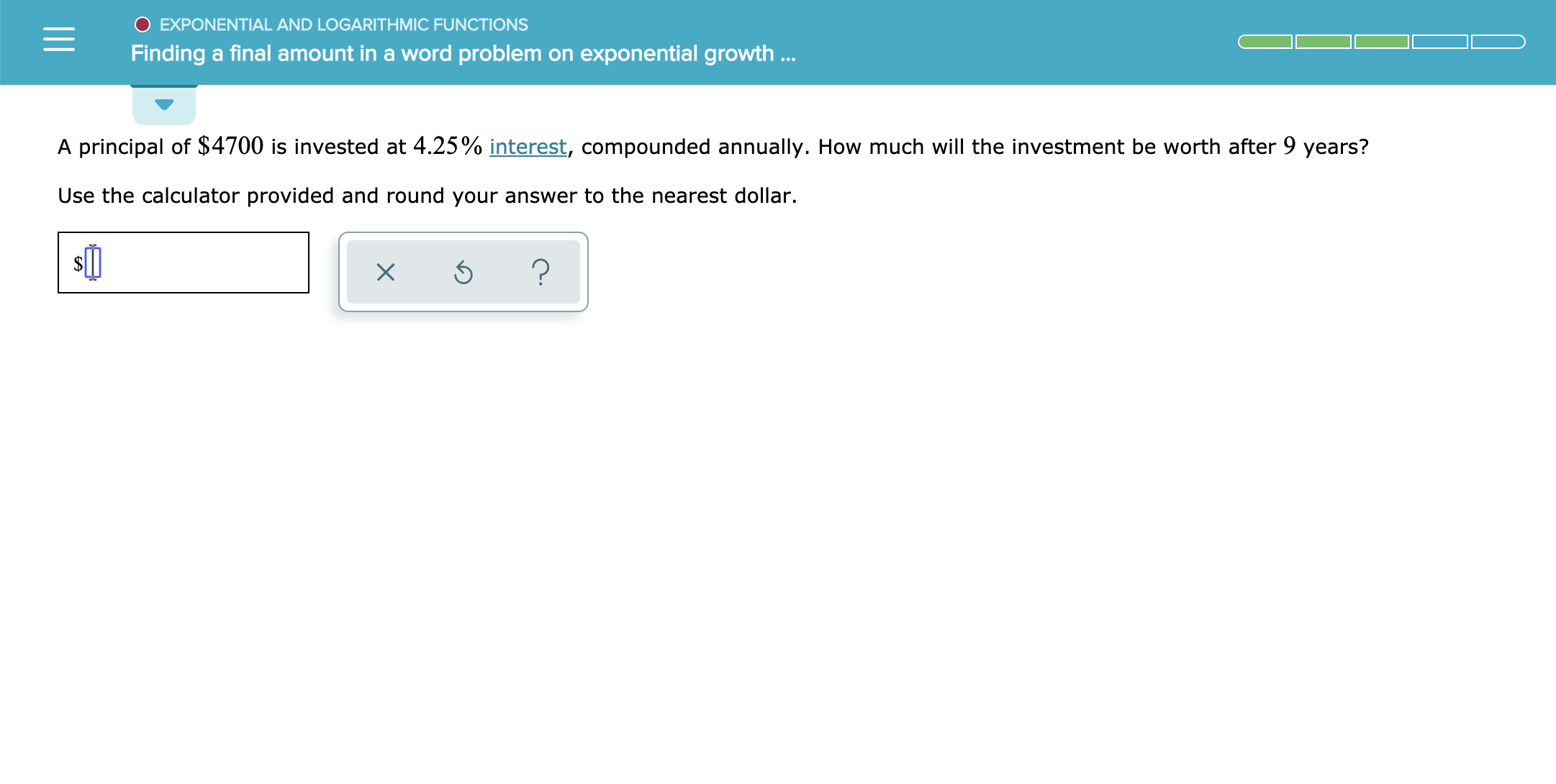O EXPONENTIAL AND LOGARITH MIC FUNCTIONSFinding a final amount in a word problem on exponential growth..A principal of \$4700 is invested at 4.25% interest, compounded annually. How much will the investment be worth after 9 years?Use the calculator provided and round your answer to the nearest dollar.X

Question

see attachmenthelp_outlineImage TranscriptioncloseO EXPONENTIAL AND LOGARITH MIC FUNCTIONS Finding a final amount in a word problem on exponential growth.. A principal of \$4700 is invested at 4.25% interest, compounded annually. How much will the investment be worth after 9 years? Use the calculator provided and round your answer to the nearest dollar. X fullscreen
Step 1

Given:

The Principal amount, P = \$4,700.

Time, t = 9 years.

Rate of interest, r...

Want to see the full answer?

See Solution

Want to see this answer and more?

Our solutions are written by experts, many with advanced degrees, and available 24/7

See Solution
Tagged in

Other